Algebra 2 2-2 Guided Practice: Direct Variation
starstarstarstarstarstarstarstarstarstar
by Matthew Richardson
| 17 Questions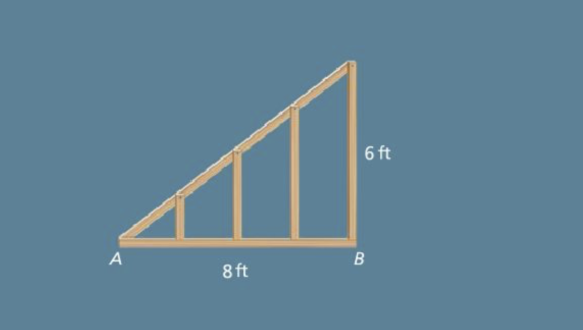1
1
10
Solve It! You are building a roof. You mark off four equal intervals from point A to point B. Then you place vertical posts as shown in the diagram. What are the heights of the four vertical posts? Select all that apply.
0.5'
1.5'
2'
3'
4.5'
5.5'
6'2
3
2
10
Take Note: Define what it means for two variables to have a direct variation relationship.
3
5
Take Note: Provide an example equation that represents a direct variation relationship.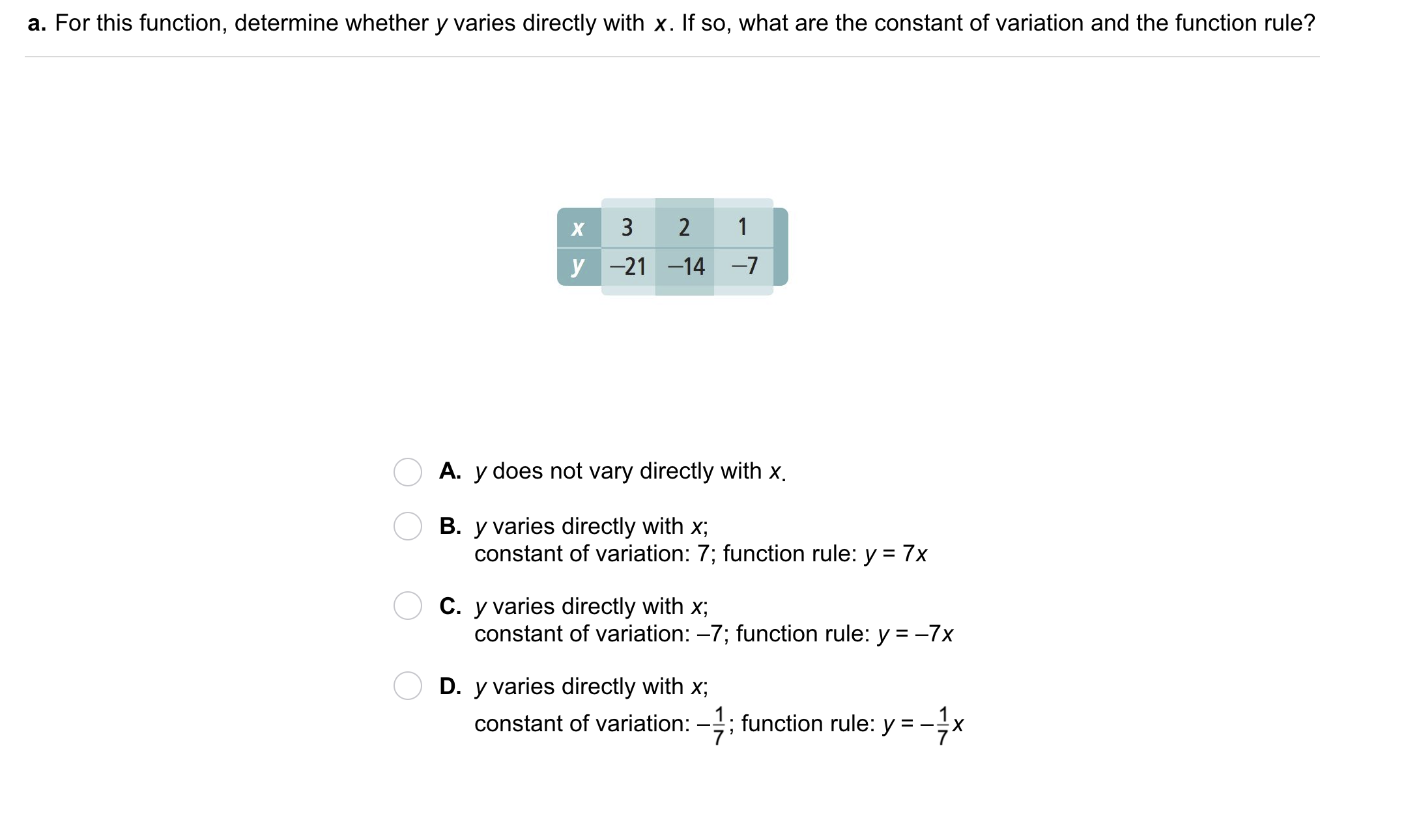4
4
10
Problem 1 Got It?
A
B
C
D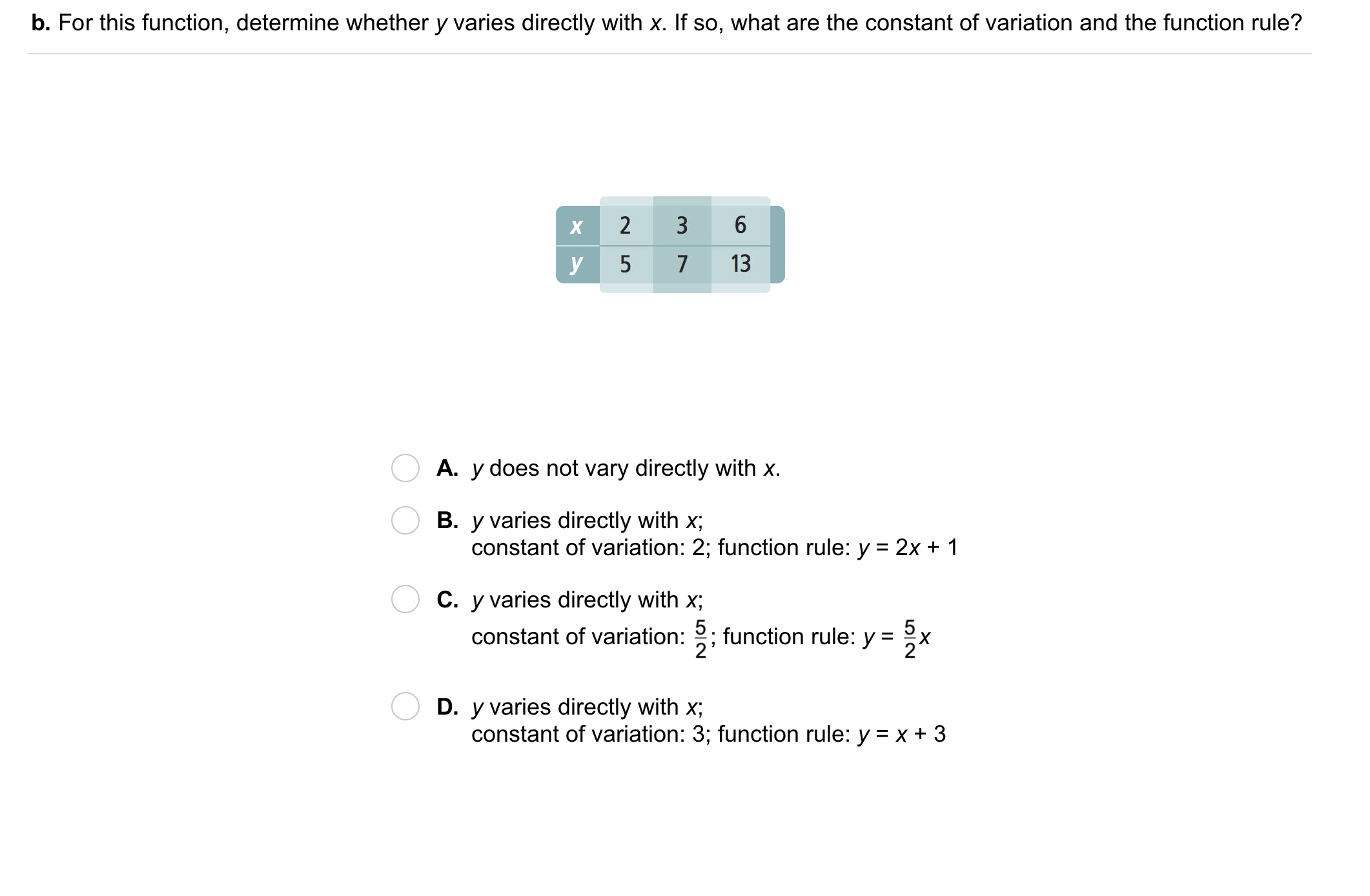5
5
10
Problem 1 Got It?
A
B
C
D6
6
10
Problem 2 Got It?
A
B
C
D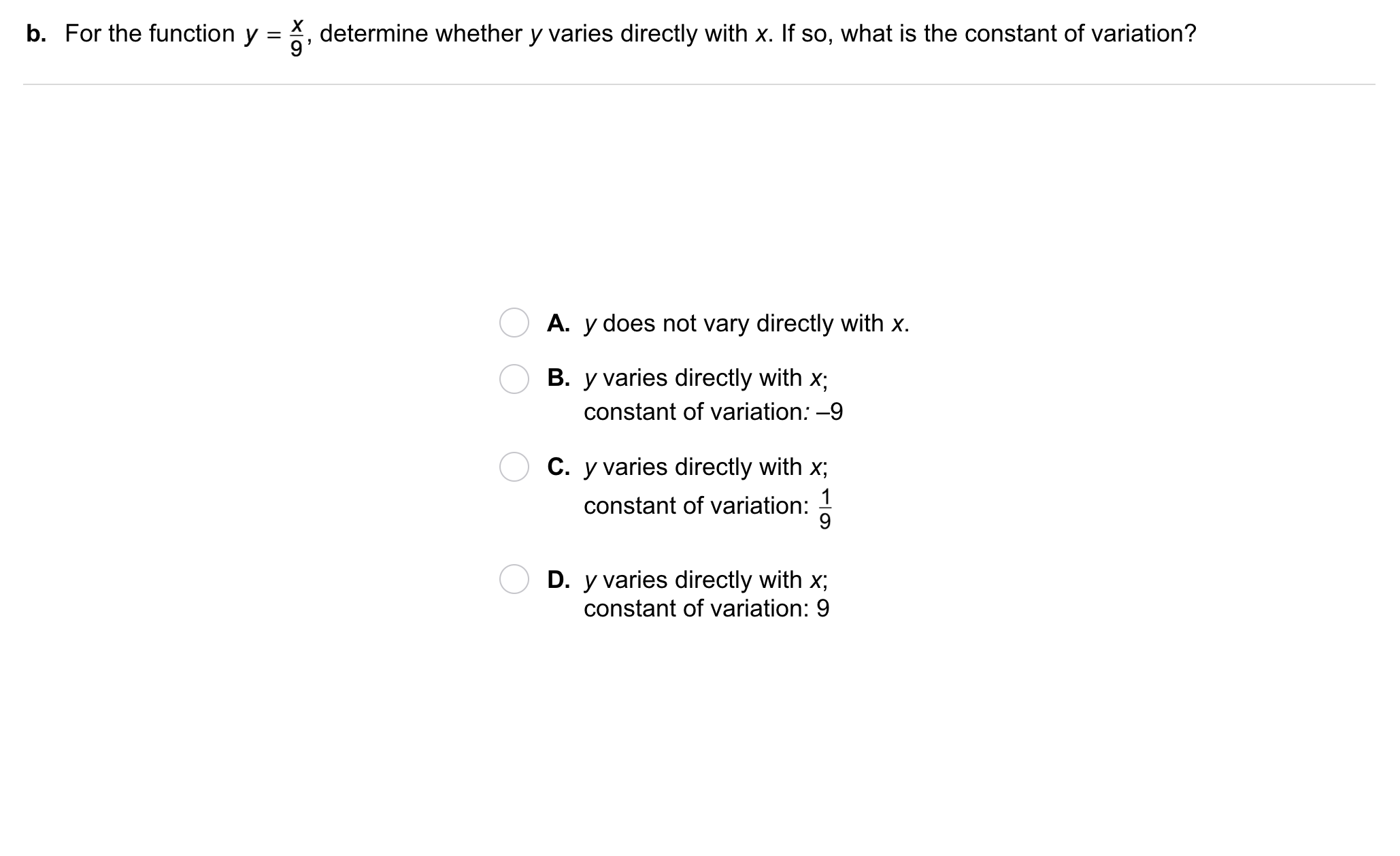7
7
10
Problem 2 Got It?
A
B
C
D8
8
10
Take Note: Describe the process of solving a direct variation using a proportion. Use the canvas, if necessary, to illustrate your explanation.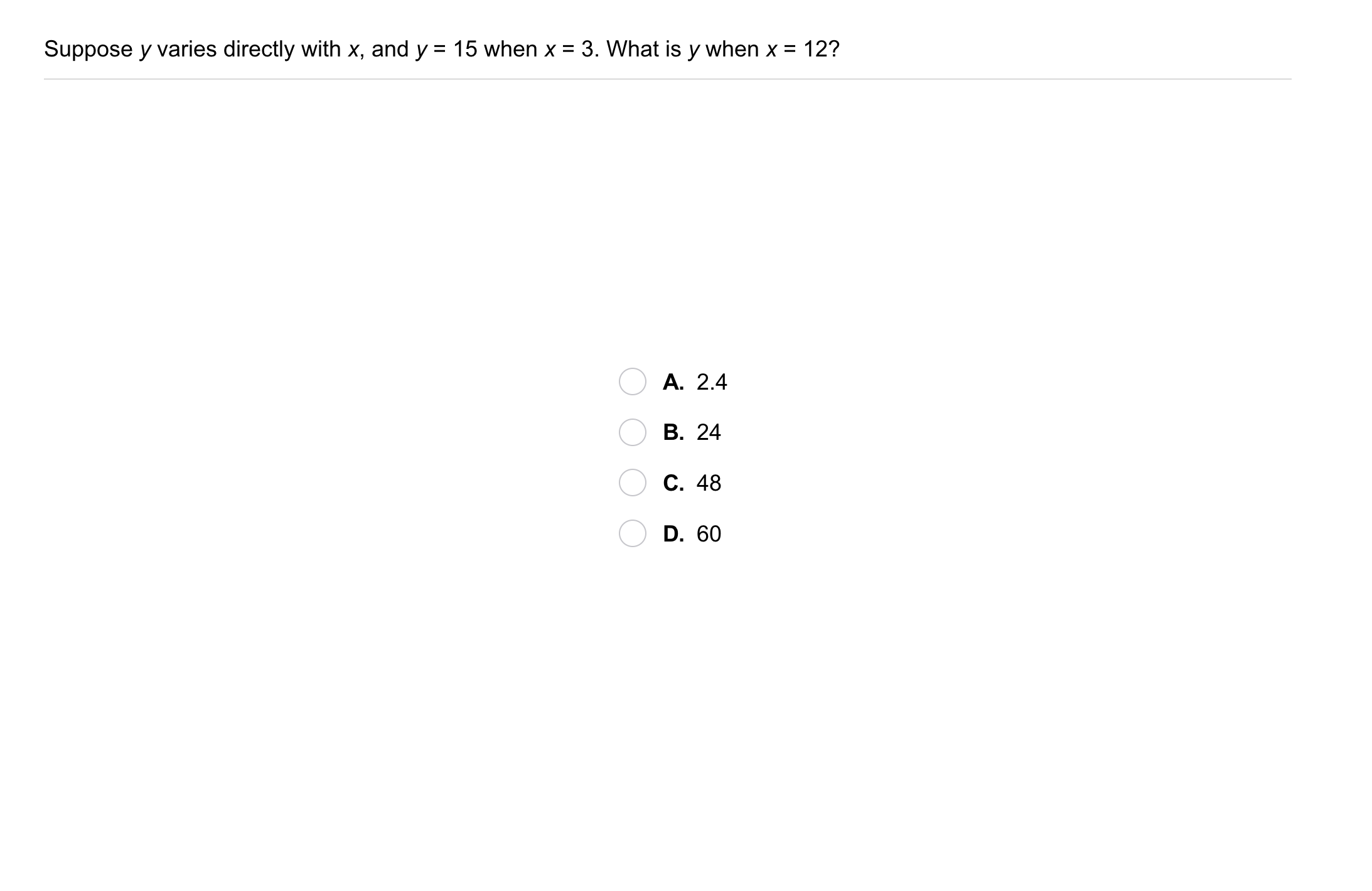9
9
10
Problem 3 Got It?
A
B
C
D
10
10
Problem 4 Got It? The number of Calories varies directly with the mass of cheese. If 50 grams of cheese contain 200 Calories, how many Calories are in 70 grams of cheese?
180 Calories
220 Calories
280 Calories
17.5 Calories
11
10
Problem 4 Got It? Reasoning: If varies directly with , does that mean y must vary directly with x?
Yes
No
12
10
Problem 4 Got It? Reasoning: Explain your response to the previous item.13
14
15
13
10
Take Note: What do all graphs of direct variation functions have in common?
14
5
Take Note: Graph an example direct variation function. Zoom and pan your graph to establish an appropriate viewing window.
15
10
Take Note: Which of the following graphs represent direct variation functions? Select all that apply.

16
20
Problem 5 Got It? Graph each direct variation equation on the canvas.17
17
10
Take Note: Summarize the mathematical content of this lesson. What topics, ideas, and vocabulary were introduced?